LARGE CHARACTERS IN BASIC

In this example I read the character rom defintions to display the characters in an enlaged shape of it.

``` 100 printchr\$(147):dim cr(5) 110 poke56334,peek(56334)and254:poke 1,peek(1)and251 120 mg\$=" just":gosub 200:mg\$="for":gosub 200:mg\$=" the":gosub 200 121 mg\$="fun":gosub 200:mg\$="of it":gosub 200 130 poke 1,peek(1)or4:poke56334,peek(56334)or1 140 goto 110 200 fori=1tolen(mg\$):cr(i)=asc(mid\$(mg\$,i,1))and191:nexti 210 for r = 0 to 7 220 for c = 1 to len(mg\$) 230 a=peek(53248+cr©*8+r) 240 for n=7 to 0 step-1:b(n)=int(a/2^n):a=a-(b(n)*2^n):next n 250 fort=0 to 7:print mid\$(" "+chr\$(113),1+b(7-t),1);:next t 260 next c : if len(mg\$)<5 then print 270 next r : return ```

CREATING THE MAZE ALTERNATIVE

This is an alternative I created for displaying a maze on the screen.

``` 10 rem -- www.justforthefunofit.nl -- 20 rem creating a maze using a 30 rem circle method to fill the screen 40 rem -------------------------------- 50 print chr\$(147) 60 for r=1 to 24 70 for a=0 to 360 80 y=(int(r*cos(a))+12) 90 x=int(r*sin(a))+20 100 rem correcting screen bouderies 110 if y < 0 then y=0 120 if y > 24then y=24 130 if x < 0 then x=0 140 if x > 40then x=40 150 poke 1024+y*40+x,77+int(rnd(1)*2) 160 next a,r ```FLIPPING OR MIRRORING A BYTE VALUE USING BASIC CODE

One small basic program I wrote was a program to inverse or mirror the bit values of a byte. I later used this routines to mirror a sprite.

The idea is that the lowest bits of a byte are transfered to the highest bytes and visa versa. See the following image explaining this a bit more.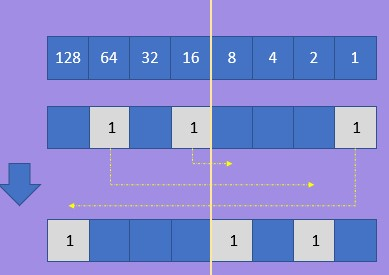If a bit is "1" in the byte then set the opposite bit in the byte to "1"
For example if the highest bit in a byte is set (value 128) then set the lowest bit (1).

I created a few different routines to accomplish this in Commodore basic.

First straightforward method (and also the fastest).

```10 b = int(rnd(1)*255) : r=. 20 if b and 1 then r=128 30 if b and 2 then r=r+64 40 if b and 4 then r=r+32 50 if b and 8 then r=r+16 60 if b and 16 then r=r+8 70 if b and 32 then r=r+4 80 if b and 64 then r=r+2 90 if b and 128 then r = r +1 100 print "reversed :" r ```

Second method using the "power to" operator and a loop.

```10 b = int(rnd(1)*255) : for n=0to7 20 if b and 2^n then r = r+2^(7-n) 30 next : print "reversed :" r , r\$```

This code is very short, however the method is quite slow on a Commodore.

And a third method doing the same but this time using a string representation en mid\$ function.

```10 b=int(rnd(1)*255) 15 r=. :b\$="00000000":r\$="00000000" 20 if b and 1 then r=128:r\$="1"+mid\$(r\$,2,8) 30 if b and 2 then r=r+64:r\$=mid\$(r\$,1,1)+"1"+mid\$(r\$,3,8) 40 if b and 4 then r=r+32:r\$=mid\$(r\$,1,2)+"1"+mid\$(r\$,4,8) 50 if b and 8 then r=r+16:r\$=mid\$(r\$,1,3)+"1"+mid\$(r\$,5,8) 60 if b and 16 then r=r+8:r\$=mid\$(r\$,1,4)+"1"+mid\$(r\$,6,8) 70 if b and 32 then r=r+4:r\$=mid\$(r\$,1,5)+"1"+mid\$(r\$,7,8) 80 if b and 64 then r=r+2:r\$=mid\$(r\$,1,6)+"1"+mid\$(r\$,8,8) 90 if b and 128 thenr=r+1: r\$=mid\$(r\$,1,7)+"1" 100 print "reversed :" r , r\$```

BASIC CODE - BREAKOUT

This time a minimalist version of the well-known game Breakout. I set myself the goal to see whether it is possible to make this in a maximum of ten lines Commodore Basic. Of course, it will not be a full version just a nice try. I wanted to have several minimal game elements in it such as:

* Colour bars
* A bouncing ball
* A paddle to bounce the ball
* Something for keeping score

It is precisely with these components that I have built up the program step by step. Below first the long version with comments and not shortened commands so that it remains readable.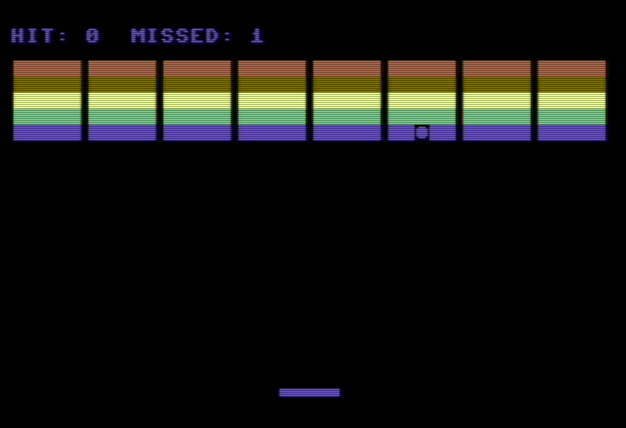To move the paddle the key "1" (left) en "2) right can be used.

```100 rem --------------------------------------------- 110 rem initialization of variables 120 rem --------------------------------------------- 130 pp=17: rem paddle start line on screen 140 sm=1024: rem screen memory start 150 sa=sm+(22*40): rem screen boundary adress range 160 dx=1:dy=1: rem initial direction of bouncing ball (positive) 170 x=int(rnd(1)*24):y=12: rem ball start position with random x position 180 c\$=chr\$(28)+chr\$(149)+chr\$(158)+chr\$(30)+chr\$(31) : rem define color values 185 1 = 1 : rem used to reverse ball on missed and last key direction 188 h = 0 : rem hit score variable 190 rem --------------------------------------------- 200 rem initialisation of screen start and color 210 rem --------------------------------------------- 220 poke 650,128 : rem set key input on repeative 230 poke 53280,0 : rem screen border color to black 240 poke 53281,0 : rem screen color to black 250 print chr\$(147): rem clear screen and cursor to top 260 rem ---------------------------------------------- 270 rem draw five color lines with eight bars 280 rem ---------------------------------------------- 290 print : rem print blanco screen line on top 300 for p=1 to 5: 310 for n=1 to 8 320 print chr\$(18)+mid\$(c\$,p,1)+chr\$(229)+" "+chr\$(234); 330 next n 340 next p 350 rem ---------------------------------------------- 360 rem start main loop (paddle and bouncing ball) 370 rem ---------------------------------------------- 380 sp=sa+pp : rem calculate start position of paddle 390 rem ---------------------------------------------- 400 rem poke the paddle characters into memory on calculated position 410 rem start and end with space (32) to clear previous on movement 420 rem ---------------------------------------------- 430 pokesp,32:pokesp+1,98:pokesp+2,98:pokesp+3,98:pokesp+4,98:pokesp+5,32 440 rem ---------------------------------------------- 450 rem get input (key "1" = left, key "2" = right 460 rem ---------------------------------------------- 470 getk:pp=pp+(k=1)-(k=2) 480 rem ---------------------------------------------- 490 rem validate boundaries' to ensure new position is within play area 500 rem ---------------------------------------------- 510 if pp<-1 then pp=-1 : rem reset pebble position to min -1 520 if pp>35 then pp=35 : rem reset pebble position to max 35 530 rem ---------------------------------------------- 540 rem clear current bouncing ball position and calculate new 550 rem ---------------------------------------------- 560 pokesm+x+(y*40),32: 570 if y>=24 or y<1 then dy=-dy : rem reverse y on boundary 580 if y>=24 then ms=ms+1 : rem count missed if y below pebble 590 if x>=39 or x<1 then dx=-dx : rem reverse x on boundary 600 if k=1 then mc=-mc : rem direction correction on hit and last key 610 x= x+dx : y= y+dy : rem new ball x,y coordinate 620 rem ---------------------------------------------- 630 rem validate hit pebble on hit count and shift to pebble position 640 rem ---------------------------------------------- 650 if peek(sm+x+(y*40))=98 then dx=-dx*mc:dy=-dy:h=h+1:x=pp 660 poke sm+x+(y*40),81 : rem poke new ball position on screen 670 print chr\$(19)"hit:";h;" missed:";ms : rem rewrite score on screen 680 goto 380 : rem restart main loop ```

BOUNCING BALLS - COMMODORE BASIC

2022-01 - This time a real classic Commodore Basic miniature "The bouncing ball". I think this classic is one of the Basic programs that when I first saw a version of it, I think almost 38 years ago (mmm am I really that …), I wanted to know how a ball could just bounce around. As a small puzzle moment today I tried to recreate a version of it as I remember it, see the resultFirst let me show the code for the most classic the single ball bouncing on the screen from side to side. I tried to keep the code clean and simple.

First version:```10 printchr\$(147):poke214,24:print:poke211,10:print"single bouncing ball" 20 sm = 1024:x=int(rnd(1)*24):y=1:dy=1:dx=1 30 poke sm+x+(y*40), 32 40 if y>=22 or y < 1 then dy=dy*-1 50 if x>=39 or x < 1 then dx=dx*-1 60 x=x+(1*dx):y=y+(1*dy):poke sm+x+(y*40),81:fors=1to100:next: goto 30```

Second version: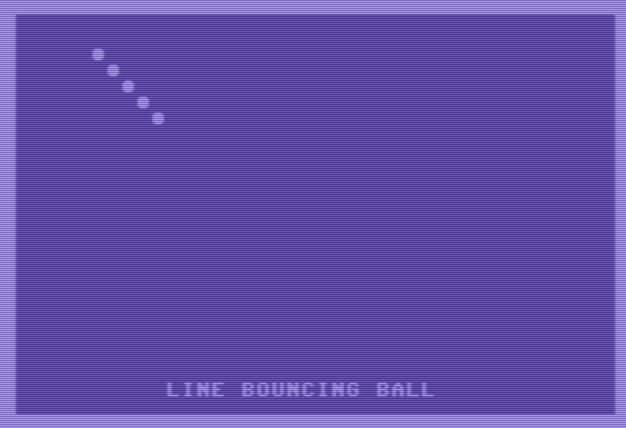```10 printchr\$(147):poke214,24:print:poke211,10:print"line bouncing ball" 20 sm = 1024:x=int(rnd(1)*24):y=1:dy=1:dx=1 30 if y>=22 or y < 1 then dy=dy*-1 40 if x>=39 or x < 1 then dx=dx*-1 50 x=x+(1*dx):y=y+(1*dy):poke sm+x+(y*40), 81 :fors=1to100:next: goto 30```

Third Version: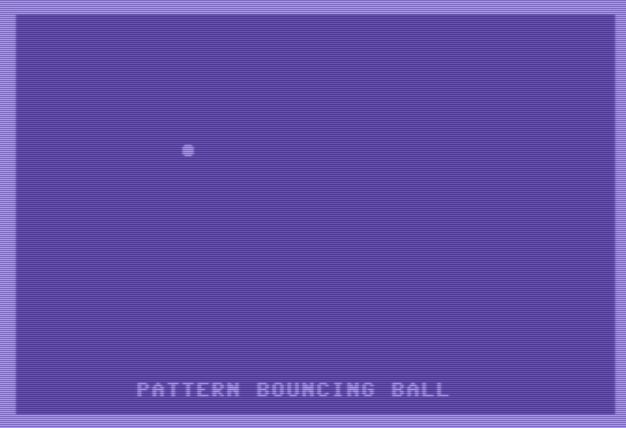```10 printchr\$(147):poke214,24:print:poke211,8:print"pattern bouncing ball" 20 sm = 1024:x=int(rnd(1)*24):y=1:dy=1:dx=1 30 poke 55296 +x+(y*40), 5+dy : rem swap between green en screen blue 40 if y>=22 or y < 1 then dy=dy*-1 50 if x>=39 or x < 1 then dx=dx*-1 60 x=x+(1*dx):y=y+(1*dy):poke sm+x+(y*40), 81 :fors=1to50:next: goto 30```

BASICCODE GAME - PATTERN MATCHING

2022 - After working on different oneliner programs I wanted to create a small basic game. The challange is to find an idea that is not to complex but also has some challanges and also a simple game concept.

At first I was just playing with some code to see how I could draw some simple rectangles on the screen. Then an idea struck me. The idea was simple and as follows:

can I create blocks with a certain pattern on the screen for which a user should search for equal patterns.
From this idea i doodled a bit on my ipad and brought it back to the following

generate a matrix of a number of rectangles on the screen let say 16
use a character that has a pattern
randomize the color of the characters and ensure they are all different
then select two random blocks in this matrix
highlight the second block
copy the pattern from the first to the second (just the color) (the second because otherwise changing color would disclose both blocks (because proces is probably to slow)
Ok next start programming, testing and try to keep it as lean and mean as possible, so first the consept nog input, output, any user input or score.

Then the result:Then the user can use see which block and pattern is equal to the one marked. After pressing a key the aswer is revealed.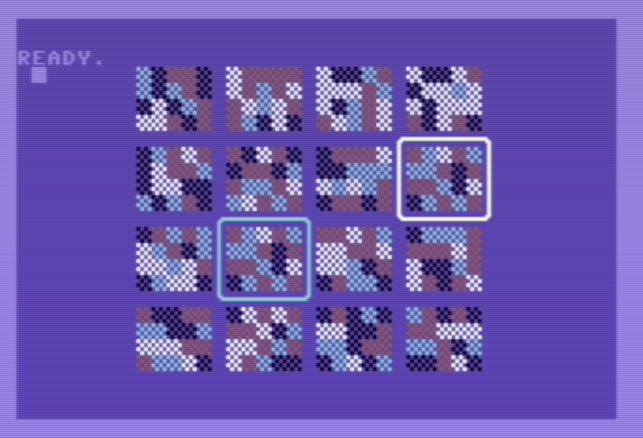Then the game code. This code can be copied directly into the VICE Commodore 64 emulator if you want to playu with it a bit.

``` 10 rem pattern matching concept game 20 rem initialize size and start position 30 dim bp(16) 35 printchr\$(147):ai=1:sm=1024:cm=55296:r=4:c=4:w=4:h=3:sp=(6-w)+((6-h)*40) 50 rem draw a matrix of random blocks 100 for b=0 to r-1:for i=1 to c:for l=0 to h:for p=0 to w 130 o =(i*(w+2))+sp+p+(40*l)+(40*b*(h+2)):pOsm+o,102:pOcm+o,int(rnd(1)*4) 150 next p,l:bp(ai)=o:ai=ai+1:next i,b 200 rem random selections of which blocks to equalize 210 f=int(rnd(1)*16)+1: s=int(rnd(1)*16)+1:if f=s then goto 210 250 rem mark first block 270 tl=f:gosub 800:co=1:rem gosub 400 :rem only for debug 280 rem copy color to second block and mark block 310 tl=s:gosub 800:co=3:gosub 400 :gosub 600 320 rem end of program 325 poke214,22:print:poke211,5:print"press a key to see the answer" 340 poke 198,0: wait 198,1 : rem wait on key pressed 350 rem disclosure other block 360 tl=f:gosub 800:co=1:gosub 400 362 poke214,22:print:poke211,5:print"press a key to play again" 365 poke 198,0: wait 198,1: goto 35 :rem again again 370 end 400 rem mark a tile use passed tile id 410 for m=0 to w+2:pokesm+lc-1+m,67:pokecm+lc-1+m,co:next m 420 for m=0 to w+2:pokesm+bc-1+m,67:pokecm+bc-1+m,co:next m 430 for m=0 to h+2:pokesm+lc-1+(m*40),66:pokecm+lc-1+(m*40),co:next m 440 for m=0 to h+2:pokesm+lc+w+1+(m*40),66:pokecm+lc+w+1+(m*40),co:next m 450 pokesm+lc-1,85:pokesm+bc-1,74:pokesm+lc+w+1,73:pokesm+bc+w+1,75 500 return 600 rem copy tile colours to from first to second tile 610 tl=f:gosub 800:fc=lc:tl=s:gosub 800 620 for i=1 to h+1:for j=0 to w: poke cm+lc+j+(i*40),peek(cm+fc+j+(i*40)) 650 next j,i : return 800 rem set determine left corner en bottom corner 810 lc = (bp(tl)-((h+1)*40)-w): rem left corner top 820 bc = (bp(tl)-w)+40: rem bottom corner 830 return```

BASIC CODE - GEOMETRIC PATTERNS

This I found on the internet ( here) from basic-fieserwolf (Needed to google a bit how to type the "Pi" character in Vice. (ctrl+page-down, in low character mode) just that you know it. So you can shorten it to one-line is you use the Petscii characters.

Here the code using the chr\$ values instead of the petscii chars to be able to show it here.

```1forx=0to39:a=cos(x/3.14/2)+cos(y/3.14/2)and15 2 poke646,a+9:printchr\$(18)+chr\$(a+190); :next: y=y+1:goto1```

BASIC CODE - CHRISTMAS TREE

Just a for the fun of it. This is one of the first basic lines that I type on the commodore after starting it up after almost 40 years.

```1?chr\$(147):k\$="":b\$="":s\$=" ":fort=1to10:k\$=k\$+"*":b\$=b\$+"**" 2?s\$+k\$:?left\$(s\$,len(s\$)-t)+b\$:next t:?left\$(s\$,len(s\$)-1)+"***"```

BASIC CODE - DRAW SINUS WITH CHARACTERS

I created this oneliner after I saw a demo on a plot function added to the Basic interpreter. I was thinking this is something I needed to try just using the standard functions.

To have more understanding on the oneliner I also have the more readable version here that can be copied directly into the vice commodore emulator if you want.

```10 for n=0 to 3*3.1415step0.05 20 x=int(4.2*n):y=int(12*sin(n)) 25 o=y*40+x+(12*40):poke1024+o,81:poke55296+o,1 30 next n```

The actual one-line miniature Basic code as typed in on the commodore could just fit in oneline using the petcii characters.

BASIC CODE - LASER BARS

This oneliner shows the use of the screen memory and color memory in combination with the poke command to put a character and color on a certain location on the screen. The position is calculated from top to bottom. This is all done in just one single line of code. This is done by entering the commands using the shortcodes as mentioned before.

`1fOc=0to39:l=rN(1)*24+1:fOx=0tol:pO1984+c-40*x,97:pO56256+c-40*x,l:nEx,c:gO1`

BASIC CODE - SNAKE USING PETCII CHARACTERS

I just played a bit with the Petscii cursor characters and the random function. The below code can be shorted using the Petscii characters, but for ease of copy here using the chr values.

This can be done in one-line, however here I will show the code using chrs\$ command for readbility. If you replace them with the Petcii coresponding characters you can fit this on one line.

```0 c\$=chr\$(145)+chr\$(157)+chr\$(29)+chr\$(17):print chr\$(147) 1 printchr\$(113)+chr\$(157);:fort=1to50:next:poke646,rnd(.)*256 2 print chr\$(18)+" "+chr\$(146)+chr\$(157)+mid\$(c\$,rnd(1)*4+1,1);:goto1```

BASIC CODE - MAZE ONELINER

This is I think one of the most known one-liner. It even has a real book written about it. This can be found here ( https://10print.org/)

`10 print chr\$(109+int(rnd(1)*2));:goto 10`

BASIC CODE - PIXELSCROLL COMMODORE 64 SCREEN

A small demontrstration to show a use of the wait command and the register 53265 to create a pixel scroll.

```10 ?chr\$(147):poke53280,6:poke53281,1:for l=1024to2023:pokel,123:nextl 30 forl=0to7:wait53265,128:poke53265,(peek(53265)and240)or7-l:poke53270,l:next 40 goto 30```

BASIC CODE - SIMPLE DEMONSTRATION OF COLOR BARS

Just showing the 16 colors of the Commodore 64 using the print and cursor position poke commands.

`10forx=.to15:poke646,x:fory=.to39:printchr\$(18)+" ";:nexty,x`

BASIC CODE - TEXT SCROLLER

This small two liner uses the poke commands to position the cursor on a certain row and line in the tekst screen.

```10 ?chr\$(147);:r=10:poke214,r:?:poke211,0:?"just for the fun of it" 20 poke1103+(r*40),peek(1064+(r*40)):poke214,r:?:poke211,1:?chr\$(20) 40 for j=0to50:next:goto 20```

BASIC CODE - COLOR BORDERS

This small basic code program for the Commodore 64 will show multicolor borders. You can copy the code below if you want to test it in Vice the commodore 64 emulator.

`0 q=53265:w=128:b=53280:wAq,w:pOb,1:pOb,2:pOb,3:pOb,4:pOb,5:pOb,6:gO0`

BASIC CODE - A NICE FLOWER

This miniature Basic program is defined using two basic code lines. I started with a one-liner only could not fit the code to realize random colorization in one line .

```1?cH(147):fOr=1to12:fOa=0to360:o=(int(r*cos(a))+12)*40+int(r*sI(a))+20 2:pO1024+o,81:pO55296+o,r:nEa,r:wait653,2```

BASIC CODE - A SMALL 10 LINE CODE PROGRAM

Iinspired" on the Modriaan painting i created this small basic program using the random function and some line math. Making use of the positioning cursor row and column on screen poke.

```10 ?chr\$(147):c\$="026":poke53281,1:poke53280,0 15 s\$=chr\$(161)+chr\$(162)+chr\$(169)+chr\$(182)+chr\$(127)+" " 20 poke214,(rnd(.)*22):?:poke211,(rnd(1)*40):?mid\$(s\$,int(rnd(1)*6+1),1) 30 poke646,val(mid\$(c\$,int(rnd(1)*3+1),1)): goto 20```

BASIC CODE - FULL ARCADE IN A ONELINER

This one is really special. I had heard of it but could not find the actual source of it until recently I discovered that it had his own web-site with a very appropriate name, namely: oneliner64.com.

It is a real one-liner fully playable game with stars and spaceship (ok, you need some imagination). You control the ship using the number "1" and "9" keys. I first tried it in Vice, but to be able to play you need to slowdown the game a bit, it is to fast.

It uses every basic abbreviation that is possible. You can read on this here. It took me a few moments to figure them out to see what I needed to type in to get it working.

This is really a master-piece of work.

`1?tArN(0)*39)"*":gEk:p=p+(k=1)-(k=9):pO1284+p,22:onp<420aN(pE(1324+p)=42)+2gO,1`

BASIC CODE - BIRDS CAN FLY ONELINER

Using different techniques a nice oneliner is created here. You can move the bird from left to right using the keys 1 and 2. In de code below ensure that the "S" in the last part is actually the clear screen ({shift{+{home} keys).

`0gEk:p=p+(k=1)-(k=2):pO214,10:?:pO211,20+p:?mI("JWKUWI",x+1,3):x=3-x:?"S":gO0`

This here is the code as can be used to copy and past into the Vice emulator. The uppercase characters will then be transfered into the PETCII characters of the commodore.

MAKING WAVES - ONLINER

One of the early classics when writing one-line basic programs on the Commodore is this one. Just making use of the Commodore key graphics to create some illusion of movement.

`10 print "------------"; :goto 10`